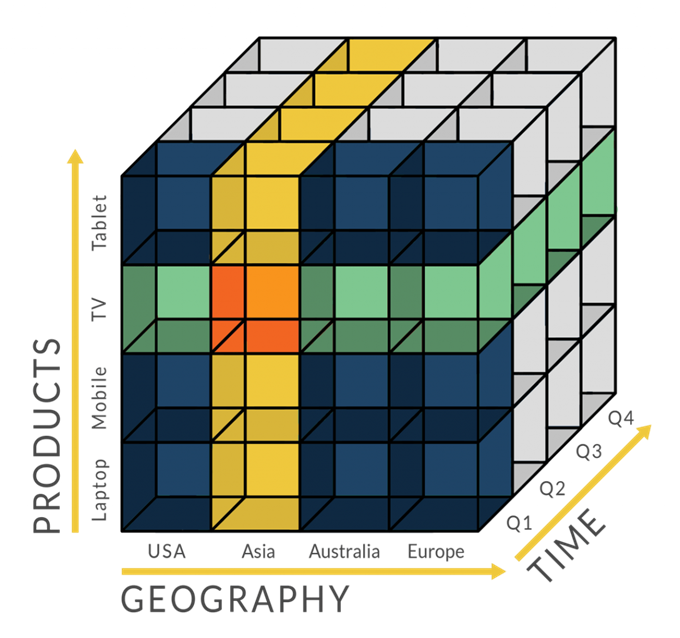# 机器学习之类别特征处理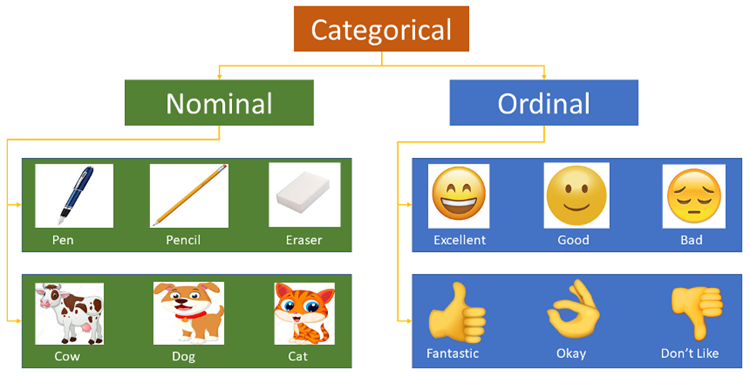## Label encoding

Label Encoding是使用字典的方式，将每个类别标签与不断增加的整数相关联，即生成一个名为class_的实例数组的索引。

Scikit-learn中的LabelEncoder是用来对分类型特征值进行编码，即对不连续的数值或文本进行编码。其中包含以下常用方法：

• fit(y) ：fit可看做一本空字典，y可看作要塞到字典中的词。
• fit_transform(y)：相当于先进行fit再进行transform，即把y塞到字典中去以后再进行transform得到索引值。
• inverse_transform(y)：根据索引值y获得原始数据。
• transform(y) ：将y转变成索引值。
from sklearn.preprocessing import LabelEncoder

le = LabelEncoder()
city_list = ["paris", "paris", "tokyo", "amsterdam"]
le.fit(city_list)
print(le.classes_)  # 输出为：['amsterdam' 'paris' 'tokyo']

city_list_le = le.transform(city_list)  # 进行Encode
print(city_list_le)  # 输出为：[1 1 2 0]

city_list_new = le.inverse_transform(city_list_le)  # 进行decode
print(city_list_new) # 输出为：['paris' 'paris' 'tokyo' 'amsterdam']


import pandas as pd
from sklearn.preprocessing import LabelEncoder

df = pd.DataFrame({
'pets': ['cat', 'dog', 'cat', 'monkey', 'dog', 'dog'],
'owner': ['Champ', 'Ron', 'Brick', 'Champ', 'Veronica', 'Ron'],
'location': ['San_Diego', 'New_York', 'New_York', 'San_Diego', 'San_Diego',
'New_York']
})

d = {}
le = LabelEncoder()
cols_to_encode = ['pets', 'owner', 'location']
for col in cols_to_encode:
df_train[col] = le.fit_transform(df_train[col])
d[col] = le.classes_


Pandas的factorize()可以将Series中的标称型数据映射称为一组数字，相同的标称型映射为相同的数字。factorize函数的返回值是一个tuple（元组），元组中包含两个元素。第一个元素是一个array，其中的元素是标称型元素映射为的数字；第二个元素是Index类型，其中的元素是所有标称型元素，没有重复。

import numpy as np
import pandas as pd

df = pd.DataFrame(['green','bule','red','bule','green'],columns=['color'])

pd.factorize(df['color'])  #(array([0, 1, 2, 1, 0], dtype=int64),Index(['green', 'bule', 'red'], dtype='object'))
pd.factorize(df['color']) #array([0, 1, 2, 1, 0], dtype=int64)
pd.factorize(df['color'])  #Index(['green', 'bule', 'red'], dtype='object')


Label Encoding只是将文本转化为数值，并没有解决文本特征的问题：所有的标签都变成了数字，算法模型直接将根据其距离来考虑相似的数字，而不考虑标签的具体含义。使用该方法处理后的数据适合支持类别性质的算法模型，如LightGBM

## 序列编码（Ordinal Encoding）

Ordinal Encoding即最为简单的一种思路，对于一个具有m个category的Feature，我们将其对应地映射到 [0,m-1] 的整数。当然 Ordinal Encoding 更适用于 Ordinal Feature，即各个特征有内在的顺序。例如对于”学历”这样的类别，”学士”、”硕士”、”博士” 可以很自然地编码成 [0,2]，因为它们内在就含有这样的逻辑顺序。但如果对于“颜色”这样的类别，“蓝色”、“绿色”、“红色”分别编码成[0,2]是不合理的，因为我们并没有理由认为“蓝色”和“绿色”的差距比“蓝色”和“红色”的差距对于特征的影响是不同的。

ord_map = {'Gen 1': 1, 'Gen 2': 2, 'Gen 3': 3, 'Gen 4': 4, 'Gen 5': 5, 'Gen 6': 6}
df['GenerationLabel'] = df['Generation'].map(gord_map)


## 独热编码(One-Hot Encoding)

• 性别：[“male”，”female”]
• 地区：[“Europe”，”US”，”Asia”]
• 浏览器：[“Firefox”，”Chrome”，”Safari”，”Internet Explorer”]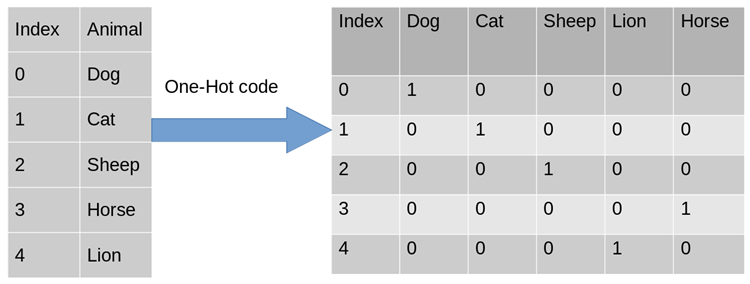• 使用one-hot编码，将离散特征的取值扩展到了欧式空间，离散特征的某个取值就对应欧式空间的某个点。在回归，分类，聚类等机器学习算法中，特征之间距离的计算或相似度的计算是非常重要的，而我们常用的距离或相似度的计算都是在欧式空间的相似度计算，计算余弦相似性，也是基于的欧式空间。
• 将离散型特征使用one-hot编码，可以会让特征之间的距离计算更加合理。比如，有一个离散型特征，代表工作类型，该离散型特征，共有三个取值，不使用one-hot编码，计算出来的特征的距离是不合理。那如果使用one-hot编码，显得更合理。

• 优点：独热编码解决了分类器不好处理属性数据的问题，在一定程度上也起到了扩充特征的作用。它的值只有0和1，不同的类型存储在垂直的空间。
• 缺点：当类别的数量很多时，特征空间会变得非常大。在这种情况下，一般可以用PCA（主成分分析）来减少维度。而且One-Hot Encoding+PCA这种组合在实际中也非常有用。

One-Hot Encoding的使用场景

• 独热编码用来解决类别型数据的离散值问题。将离散型特征进行one-hot编码的作用，是为了让距离计算更合理，但如果特征是离散的，并且不用one-hot编码就可以很合理的计算出距离，那么就没必要进行one-hot编码，比如，该离散特征共有1000个取值，我们分成两组，分别是400和600,两个小组之间的距离有合适的定义，组内的距离也有合适的定义，那就没必要用one-hot 编码。
• 基于树的方法是不需要进行特征的归一化，例如随机森林，bagging 和 boosting等。对于决策树来说，one-hot的本质是增加树的深度，决策树是没有特征大小的概念的，只有特征处于他分布的哪一部分的概念。

LabelBinarizer：将对应的数据转换为二进制型，类似于onehot编码，这里有几点不同：

• 可以处理数值型和类别型数据
• 输入必须为1D数组
• 可以自己设置正类和父类的表示方式
from sklearn.preprocessing import LabelBinarizer

lb = LabelBinarizer()

city_list = ["paris", "paris", "tokyo", "amsterdam"]

lb.fit(city_list)
print(lb.classes_)  # 输出为：['amsterdam' 'paris' 'tokyo']

city_list_le = lb.transform(city_list)  # 进行Encode
print(city_list_le)  # 输出为：
# [[0 1 0]
#  [0 1 0]
#  [0 0 1]
#  [1 0 0]]

city_list_new = lb.inverse_transform(city_list_le)  # 进行decode
print(city_list_new)  # 输出为：['paris' 'paris' 'tokyo' 'amsterdam']


OneHotEncoder只能对数值型数据进行处理，需要先将文本转化为数值（Label encoding）后才能使用，只接受2D数组：

import pandas as pd
from sklearn.preprocessing import LabelEncoder
from sklearn.preprocessing import OneHotEncoder

def LabelOneHotEncoder(data, categorical_features):
d_num = np.array([])
for f in data.columns:
if f in categorical_features:
le, ohe = LabelEncoder(), OneHotEncoder()
data[f] = le.fit_transform(data[f])
if len(d_num) == 0:
d_num = np.array(ohe.fit_transform(data[[f]]))
else:
d_num = np.hstack((d_num, ohe.fit_transform(data[[f]]).A))
else:
if len(d_num) == 0:
d_num = np.array(data[[f]])
else:
d_num = np.hstack((d_num, data[[f]]))
return d_num

df = pd.DataFrame([
['green', 'Chevrolet', 2017],
['blue', 'BMW', 2015],
['yellow', 'Lexus', 2018],
])
df.columns = ['color', 'make', 'year']

df_new = LabelOneHotEncoder(df, ['color', 'make', 'year'])


import pandas as pd

df = pd.DataFrame([
['green', 'Chevrolet', 2017],
['blue', 'BMW', 2015],
['yellow', 'Lexus', 2018],
])
df.columns = ['color', 'make', 'year']

df_processed = pd.get_dummies(df, prefix_sep="_", columns=df.columns[:-1])
print(df_processed)


get_dummies的优势在于:

• 本身就是 pandas 的模块，所以对 DataFrame 类型兼容很好
• 不管你列是数值型还是字符串型，都可以进行二值化编码
• 能够根据指令，自动生成二值化编码后的变量名

get_dummies虽然有这么多优点，但毕竟不是 sklearn 里的transformer类型，所以得到的结果得手动输入到 sklearn 里的相应模块，也无法像 sklearn 的transformer一样可以输入到pipeline中进行流程化地机器学习过程。

## 频数编码（Frequency Encoding/Count Encoding）

import pandas as pd

data_count = data.groupby('城市')['城市'].agg({'频数':'size'}).reset_index()
data = pd.merge(data, data_count, on = '城市', how = 'left')


## 目标编码（Target Encoding/Mean Encoding）

LabelEncoder能够接收不规则的特征列，并将其转化为从0到n-1的整数值（假设一共有n种不同的类别）；OneHotEncoder则能通过哑编码，制作出一个m*n的稀疏矩阵（假设数据一共有m行，具体的输出矩阵格式是否稀疏可以由sparse参数控制）。

• LabelEncoder编码高基数定性特征，虽然只需要一列，但是每个自然数都具有不同的重要意义，对于y而言线性不可分。使用简单模型，容易欠拟合（underfit），无法完全捕获不同类别之间的区别；使用复杂模型，容易在其他地方过拟合（overfit）。
• OneHotEncoder编码高基数定性特征，必然产生上万列的稀疏矩阵，易消耗大量内存和训练时间，除非算法本身有相关优化（例：SVM）。

• 增加计算时间，因为需要对每一个类别型特征，在迭代的每一步，都需要对GS进行计算
• 增加存储需求，对于一个类别型变量，需要存储每一次分离每个节点的类别

$$s = \frac{1}{1+\exp(-\frac{n-mdl}{a})}$$

$$\hat{s}^k=prior * (1-s) + s * \frac{n^+}{n}$$

• 增加正则项a的大小
• 在训练集该列中添加噪声
• 使用交叉验证

• 把train data划分为20-folds （举例：infold: fold #2-20, out of fold: fold #1）
• 将每一个 infold （fold #2-20） 再次划分为10-folds （举例：inner_infold: fold #2-10, Inner_oof: fold #1）
• 计算 10-folds的 inner out of folds值 （举例：使用inner_infold #2-10 的target的均值，来作为inner_oof #1的预测值）
• 对10个inner out of folds 值取平均，得到 inner_oof_mean
• 计算oof_mean （举例：使用 infold #2-20的inner_oof_mean 来预测 out of fold #1的oof_mean
• 将train data 的 oof_mean 映射到test data完成编码

from category_encoders import TargetEncoder
import pandas as pd

# prepare some data
y_train = bunch.target[0:250]
y_test = bunch.target[250:506]
X_train = pd.DataFrame(bunch.data[0:250], columns=bunch.feature_names)
X_test = pd.DataFrame(bunch.data[250:506], columns=bunch.feature_names)

# use target encoding to encode two categorical features

# transform the datasets
training_numeric_dataset = enc.fit_transform(X_train, y_train)
testing_numeric_dataset = enc.transform(X_test)


## Beta Target Encoding

Kaggle竞赛Avito Demand Prediction Challenge 第14名的solution分享：14th Place Solution: The Almost Golden Defenders。和target encoding 一样，beta target encoding 也采用 target mean value （among each category） 来给categorical feature做编码。不同之处在于，为了进一步减少target variable leak，beta target encoding发生在在5-fold CV内部，而不是在5-fold CV之前：

• 把train data划分为5-folds （5-fold cross validation）
• target encoding based on infold data
• train model
• get out of fold prediction

# train -> training dataframe
# test -> test dataframe
# N_min -> smoothing term, minimum sample size, if sample size is less than N_min, add up to N_min
# target_col -> target column
# cat_cols -> categorical colums
# Step 1: fill NA in train and test dataframe

# Step 2: 5-fold CV (beta target encoding within each fold)

kf = KFold(n_splits=5, shuffle=True, random_state=0)
for i, (dev_index, val_index) in enumerate(kf.split(train.index.values)):
# split data into dev set and validation set
dev = train.loc[dev_index].reset_index(drop=True)
val = train.loc[val_index].reset_index(drop=True)

feature_cols = []
for var_name in cat_cols:
feature_name = f'{var_name}_mean'
feature_cols.append(feature_name)

prior_mean = np.mean(dev[target_col])
stats = dev[[target_col, var_name]].groupby(var_name).agg(['sum', 'count'])[target_col].reset_index()

### beta target encoding by Bayesian average for dev set
df_stats = pd.merge(dev[[var_name]], stats, how='left')
df_stats['sum'].fillna(value = prior_mean, inplace = True)
df_stats['count'].fillna(value = 1.0, inplace = True)
N_prior = np.maximum(N_min - df_stats['count'].values, 0)   # prior parameters
dev[feature_name] = (prior_mean * N_prior + df_stats['sum']) / (N_prior + df_stats['count']) # Bayesian mean

### beta target encoding by Bayesian average for val set
df_stats = pd.merge(val[[var_name]], stats, how='left')
df_stats['sum'].fillna(value = prior_mean, inplace = True)
df_stats['count'].fillna(value = 1.0, inplace = True)
N_prior = np.maximum(N_min - df_stats['count'].values, 0)   # prior parameters
val[feature_name] = (prior_mean * N_prior + df_stats['sum']) / (N_prior + df_stats['count']) # Bayesian mean

### beta target encoding by Bayesian average for test set
df_stats = pd.merge(test[[var_name]], stats, how='left')
df_stats['sum'].fillna(value = prior_mean, inplace = True)
df_stats['count'].fillna(value = 1.0, inplace = True)
N_prior = np.maximum(N_min - df_stats['count'].values, 0)   # prior parameters
test[feature_name] = (prior_mean * N_prior + df_stats['sum']) / (N_prior + df_stats['count']) # Bayesian mean

# Bayesian mean is equivalent to adding N_prior data points of value prior_mean to the data set.
del df_stats, stats
# Step 3: train model (K-fold CV), get oof prediction


## M-Estimate Encoding

M-Estimate Encoding 相当于 一个简化版的Target Encoding：

$$\hat{x}^k=\frac{n^{+}+prior*m}{y^{+}+m}$$

## James-Stein Encoding

James-Stein Encoding 同样是基于target的一种算法。算法的思想很简单，对于特征的每个取值 k 可以根据下面的公式获得：

$$\hat{x}^k=(1-B)*\frac{n^+}{n}+B*\frac{y^+}{y}$$

$$B = \frac{Var[y^k]}{Var[y^k]+Var[y]}$$

## Weight of Evidence Encoder

Weight Of Evidence 同样是基于target的方法。

$$WOE_i = \log(\frac{B_i/B_{total}}{G_i/G_{total}})=\log(\frac{B_i/G_i}{B_{total}/G_{total}})$$

WOE特别合适逻辑回归，因为Logit=log(odds)。WOE编码的变量被编码为统一的维度（是一个被标准化过的值），变量之间直接比较系数即可。

## Leave-one-out Encoder (LOO or LOOE)

$$\hat{x}_i^k=\frac{\sum_{j\neq i}(y_i*(x_j==k))-y_i}{\sum_{j\neq i}x_j==k}$$

## Probability Ratio Encoding

$$PR_i = \frac{B_i/B_{total}}{G_i/G_{total}}=\frac{B_i/G_i}{B_{total}/G_{total}}$$

## Helmert Encoding

Helmert编码通常在计量经济学中使用。在Helmert编码（分类特征中的每个值对应于Helmert矩阵中的一行）之后，线性模型中编码后的变量系数可以反映在给定该类别变量某一类别值的情形下因变量的平均值与给定该类别其他类别值的情形下因变量的平均值的差值。

Helmet编码是仅次于One-Hot Encoding和Sum Encoder使用最广泛的编码方法，与Sum Encoder不同的是，它比较的是某一特征取值下对应标签（或其他相关变量）的均值与他之前特征的均值之间的差异，而不是和所有特征的均值比较。 这个特征同样容易出现过拟合的情况。

## CatBoost Encoding

• 将输入样本集随机排序，并生成多组随机排列的情况。
• 将浮点型或属性值标记转化为整数。
• 将所有的分类特征值结果都根据以下公式，转化为数值结果。

$$avgTarget = \frac{countInClass + prior}{totalCount + 1}$$

CatBoost处理Categorical features总结：

• 首先，他们会计算一些数据的statistics。计算某个category出现的频率，加上超参数，生成新的numerical features。这一策略要求同一标签数据不能排列在一起（即先全是0之后全是1这种方式），训练之前需要打乱数据集。
• 第二，使用数据的不同排列（实际上是4个）。在每一轮建立树之前，先扔一轮骰子，决定使用哪个排列来生成树。
• 第三，考虑使用categorical features的不同组合。例如颜色和种类组合起来，可以构成类似于blue dog这样的feature。当需要组合的categorical features变多时，catboost只考虑一部分combinations。在选择第一个节点时，只考虑选择一个feature，例如A。在生成第二个节点时，考虑A和任意一个categorical feature的组合，选择其中最好的。就这样使用贪心算法生成combinations。
• 第四，除非向gender这种维数很小的情况，不建议自己生成one-hot vectors，最好交给算法来处理。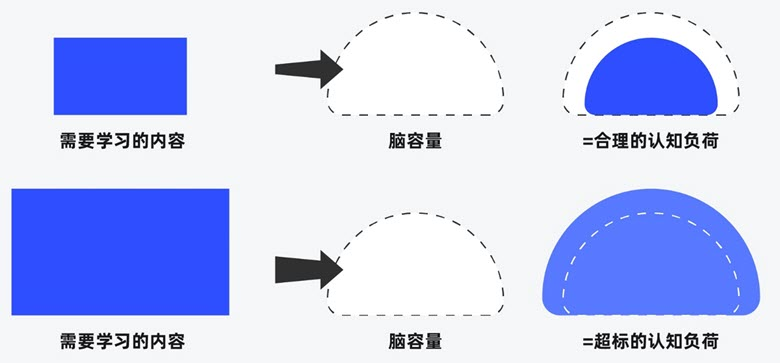##### 界面设计和游戏设计中的认知负荷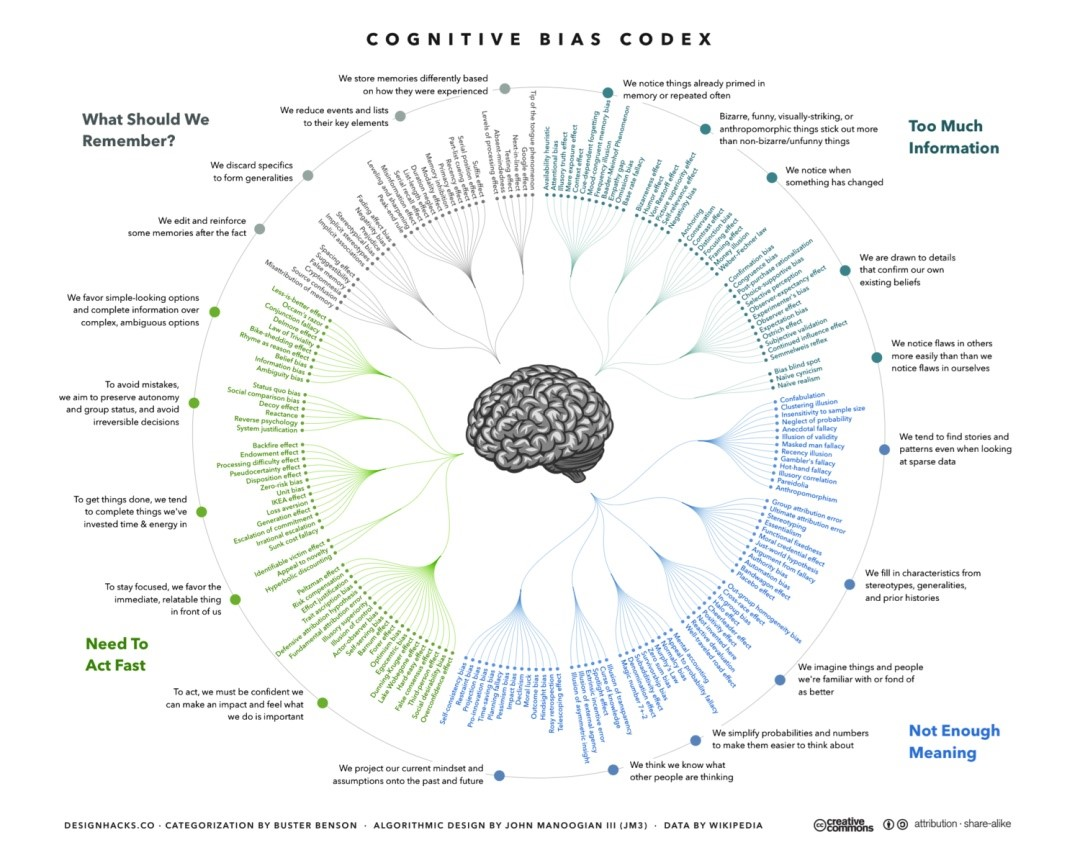##### 理解认知偏差